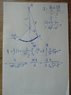# Potential and intensity of magnetic field

• ciso112
LaTeX can handle this pretty easily.It's a typsetting markup script called LaTeX - and it's pretty much the academic standard ... you can use it for Libre Office too, though, as you've noticed, there is a limited Tex support already built in.Yes, I have noticed that LaTeX has good support for complex mathematics -- and that's why I chose it.f

## Homework Statement

Very thin wire bent into the shape of a quarter-circle with the radius R is uniformly charged with electric charge q.
Calculate the potential and intensity of the electric field at point A, which lies on a line perpendicular to the plane of the semicircle and is passing through the center of its curvature at a distance a from the center. The wire is in the vacuum.

## Homework Equations

I am integrating through the length of the conductor, where all the variables seem to be the constants. Is it correct?
How would it look like if I took into account the changing of the angle?

## The Attempt at a Solution

see the picture

--
this is my first post, greetings to all of you and thanks for the help
Lukas

#### Attachments

•IMG_20160518_183559.jpg
28 KB · Views: 420
Welcome to PF.
I am integrating through the length of the conductor, where all the variables seem to be the constants. Is it correct?
Yes.
How would it look like if I took into account the changing of the angle?
You mean if you take θ anticlockwise about the axis, from one end of the wire?
Try it and see: dx = R.dθ and the limits of integration are 0 - π/2 ...

You mean if you take θ anticlockwise about the axis, from one end of the wire?
Try it and see: dx = R.dθ and the limits of integration are 0 - π/2 ...

Yes...mainly "dx = R.dθ" made the trick, thanks again. (the result is the same :) )

Now everything is ready for the second question:
I need to calculate the intensity, I was thinking of using the equation "(vector)E = - grad θ" which gives me the partial derivations by x,y,z.
But θ doesn't contain x,y or z, so I would stay only with constants and therefore 0...what am I missing?

Last edited:
##\vec E = -\nabla \phi## ... ;) where ##\phi## is the electric potential. I used ##\theta## for the angle.
How to write equations: https://www.physicsforums.com/help/latexhelp/

You have only worked out the electric potential at a specific point.
##\phi## is not the same everywhere... therefore it has a gradient someplace.
But it may be easier to do the vector calculus for the electric field directly: exploit the symmetry.

##\vec E = -\nabla \phi## ... ;) where ##\phi## is the electric potential. I used ##\theta## for the angle.
How to write equations: https://www.physicsforums.com/help/latexhelp/

I really like the logic behind, it recommends me LibreOffice and its writing of the equations.

You have only worked out the electric potential at a specific point.
##\phi## is not the same everywhere... therefore it has a gradient someplace.
But it may be easier to do the vector calculus for the electric field directly: exploit the symmetry.

Thx for the guidance, it is clearer now, hope I will do the calculations correctly.

I really like the logic behind, it recommends me LibreOffice and its writing of the equations.
It's a typsetting markup script called LaTeX - and it's pretty much the academic standard ... you can use it for Libre Office too, though, as you've noticed, there is a limited Tex support already built in.
http://extensions.libreoffice.org/extension-center/texmaths-1

Thx for the guidance, it is clearer now, hope I will do the calculations correctly.
No worries - it can help to explicitly lay out the axes ... I used cartesian with the charges in the positive quadrant of the x-y plane and A on the z axis.

•ciso112